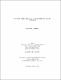## Optimal Paths Related to Discrete Transport Problems2015
##### Authors
Sultana, Sharmin
University of Lethbridge, Faculty of Arts and Science
##### Publisher
Lethbridge, Alta : University of Lethbridge, Dept. of Mathematics and Computer Science
##### Abstract
The transport problem proposed by Monge in the 1780's, was to find the best way to move a pile of soil or rubble to an excavation or fill, with the least effort where the cost of a transport map or the transport plan is generally determined by the integral of some powers of the distance, such as $\vert x-y \vert ^ p$. But in many cases in real applications, the actual cost is not generally determined by a transport path. Sometimes a Y-shaped'' path is less expensive compared to a V-shaped path'', to transport items from two starting point to one destination point. Here, we will show that one can transport any Radon probability measure to another Radon probability measure through a general optimal transport path, which is given by a vector measure in our setting. Also, we define a new distance function $d_\alpha$ on the space of probability measures which indeed metrizes the weak* topology of measures. This thesis is an exposition of a paper by Qinglan Xia, Optimal paths related to transport problems'', World Scientific, $51(2): 252-289, 2002$.
##### Keywords
Radon measure , optimal transport path